In order to show dispersion parameters of optical fiber, we perform the Taylor expansion of a mode propagation constant,β, around a center frequency,ω0. Then, the propagation constant is given as follows.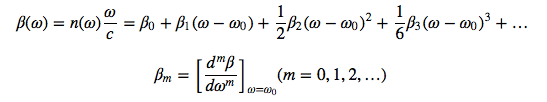The parameter β1 and β2 are expressed as a function of refractive index, n, and its derivative.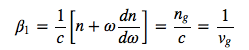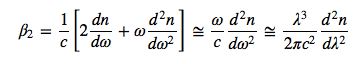In Eqs., ng is a group refractive index, and vg is a group velocity. β1=1/vg is a propagation delay time per unit length, and is called as a group delay time. β2 is GVD, which represents an elongation of pulse duration. In Fig.2.15, (a) shows the refractive index, n, and the group refractive index, ng, and (b) shows the the group velocity dispersion, β2, for bulk silica glass as a function of wavelength. λD in Fig.2.15 is the zero dispersion wavelength. For silica glass, the zero dispersion wavelength is approximately 1.27 μm. The dispersion in Fig.2.15 is derived from the refractive index of silica glass, and is called as a material dispersion. In a practical optical fiber, the core includes a slight amount of dopant such as GeO2 and P2O5, and the dispersion property depends on the concentration of dopant. For a material with the relatively larger refractive index (n), the absolute value of GVD is generally larger.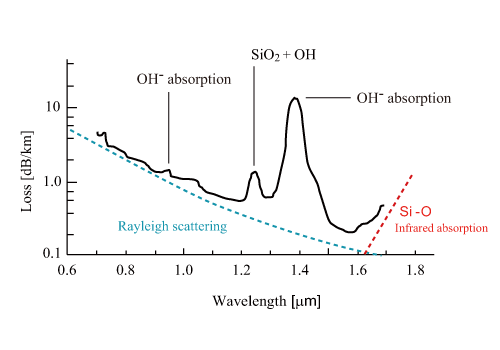Figure 2.15: Dispersion characteristics for silica glass. (a) Wavelength dependency of refractive index, n, and group refractive index, ng, of silica glass, (b) wavelength dependency of group velocity dispersion, β2. λD is zero-dispersion wavelength.

In describing a dispersion of optical fiber, a dispersion parameter, D [ps/nm/km], is employed instead of GVD, β2 [ps2/km]. A relationship between the dispersion parameter, D, and the GVD, β2, is expressed as follows.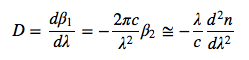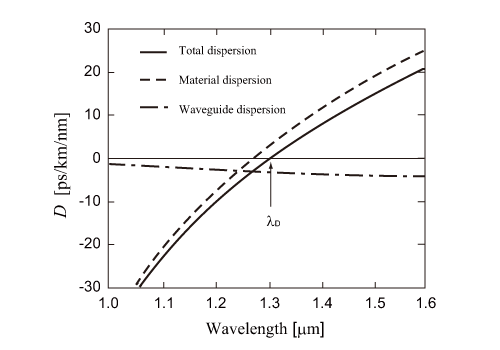Figure 2.16: Full dispersion characteristics for silica optical fiber

The total dispersion of optical fiber is the sum of material dispersion and waveguide (structure) dispersion. The waveguide dispersion depends on the core radius and the refractive index difference between the core and the clad (Δ). By calculating the material dispersion and the waveguide dispersion from the references, and by summing them up, the total dispersion is obtained as shown in Fig.. The zero dispersion wavelength, λD, for a typical optical fiber is 1.31 μm. The zero dispersion wavelength of the material dispersion is redshifted bt the waveguide dispersion.

For λ<λD, we have D0), and a fiber exhibits a normal dispersion. In the wavelength range of a normal dispersion, higher-frequency (shorter-wavelength) components in an optical pulse propagates relatively slowly compared with lower-frequency (longer-wavelength) components. On the other hand, the wavelength range of for λ>λD is called as a abnormal dispersion. The abnormal dispersion exhibits an opposite of the normal dispersion in terms of the characteristics of optical pulse propagation.

In the typical case, a dispersion indicates β2, the second order differential of β by ω. Higher-order dispersions indicate β3, the first order differential of β2 by ω, and the higher-order differential of β2 by ω. The higher-order dispersion must be considered for either a pulse in the vicinity to λ=λD or a relatively wideband pulse such as an ultrashort optical pulse with a duration of 100 fs or shorter. In using a wideband optical pulse, the fundamental, the first-order, and the second-order items in 1st Eq. are not enough for calculating a dispersion, therefore, higher-order dispersion must be considered.

Higher-order dispersions generally yield a distortion of wave shape. An optical pulse elongated by the second-order dispersion can be restored without much effort after the distorted pulse propagates through a dispersive material that cancels out the dispersion. In order to compensate the higher-order dispersions, a mode precise compensation is needed.